# Beds

At the summer camp, there are 41 chalets. Some rooms are 3-beds, some 4-beds. How many campers from 140 are living in 3-bed?

Correct result:

x =  72

#### Solution: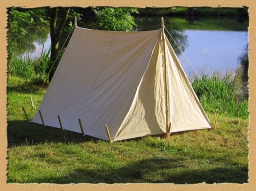We would be pleased if you find an error in the word problem, spelling mistakes, or inaccuracies and send it to us. Thank you!Tips to related online calculators
Do you have a linear equation or system of equations and looking for its solution? Or do you have quadratic equation?

## Next similar math problems:

• Cottages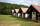The summer camp is 41 cottages. Rooms are for three and for four in them. How many of the 140 campers lives of three?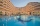Up to 48 rooms, some of which are triple and some quadruple, accommodated 173 people so that all beds are occupied. How many triple and how many quadruple rooms were there?
• HostelStudents are accommodated in 22 rooms. Rooms were 4 and 6 bed. How many rooms in which type occupied 106 children there?
• College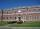At the college are at 57 rooms 194 students. Some rooms are triple and some quad. How many triple and quadruple rooms there is, if it is all fully occupied?
• Hotel roomsIn the 45 rooms, there were 169 guests, some rooms were three-bedrooms and some five-bedrooms. How many rooms were?
• CoinsThe money - coins are minted from the hardest bronze, which contains copper and tin in a ratio of 41: 9. How much copper and tin are in 2kg of bronze money?
• The dormitoryThe dormitory accommodates 150 pupils in 42 rooms, some of which are triple and some are quadruple. Determine how many rooms are triple and how many quadruples.
• Banknotes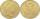$1390 was collected. How much was in$20 notes and how many in $50 notes in that order? How many solutions exists? • PharmacyAt the pharmacy are in one container 20% solution in the second 50% solution of disinfectant. They need to prepare 4 L of 48-percent solution. What amount of solution from each container is needed to mix? • Ski lessons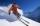On the ski course leaves 80 pupils. They live in triple and quadruple rooms. Count if rooms is the 23. How many are triple and quadruple rooms? • Tulips and daffodils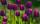Two hundred twenty tulips and daffodils are planted in the flowerbed. One-third of all tulips and one-sixth of all daffodils equals the number of all tulips. How many tulips and how many daffodils? • Tea blendTea blends are maked from two kinds of tea. In standard tea mixture are two teas in the ratio 1:3 and 40 g costs 42 CZK. In the premium tea mixture are weighing two teas in the ratio 1:1 and 50 grams costs 60 CZK. How much cost 10 grams of more expensive • Ladislav in Amsterdam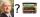Ladislav was a reward and went to a conference in Amsterdam. The conference fee was € 2536. Calculate how many books Ladislav could buy at the price of 46 and 54 euros if he doesn't want to fill the whole home library, and he can buy only 52 books? • Ľé sweetsThere are 20 sweets in the bag. Some are chocolate, other coconuts, and the remaining marzipan. Chocolate is 4 times more than coconut. Marzipan's less than chocolate. How much is in a bag of coconut sweets? • Tourist cottage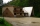The tourist cottage has 11 rooms for 16 guests, some of them are doubles, some singles. How many are double rooms and how many are single rooms? • Two math problems1) The sum of twice a number and -6 is nine more than the opposite of that number. Find the number. 2) A collection of 27 coins, all nickels, and dimes, is worth$2.10. How many of each coin are there? The dime, in United States usage, is a ten-cent coin.
• Candy and boxesWe have some number of candy and empty boxes. When we put candies in boxes of ten, there will be 2 candies and 8 empty boxes left, when of eight, there will be 6 candies and 3 boxes left. How many candy and empty boxes left when we put candies in boxes of